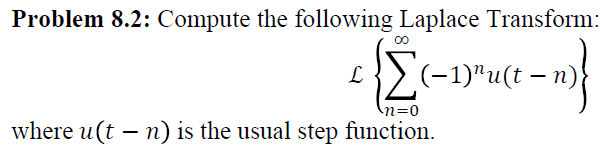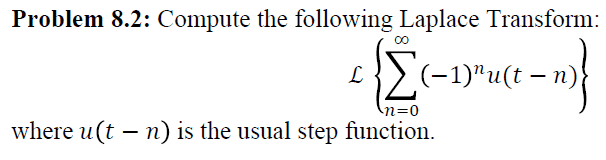# Laplace Transform help needed

Hi,

I've been asked to find the Laplace transform of a function and I have not the slightest clue where to begin. My professor derived the basic Laplace transforms in class(sin, cos, delta function, step function, etc), all of which I understood perfectly. However, he never really gave us an example of how to use those to find other Laplace transforms, let alone discuss how to approach the homework problem.i made a few attempts. The first was by saying that the transform of a summation is the summation of the transforms. And then trying to take the transform of that, which seems ugly. I also tried graphing it and re-writing it as a series of step functions to get an idea, but I obviously can't do that until the end of time, so I'm stuck. Could somebody lead me in the right direction? Thanks!

Mark44
Mentor
Hi,

I've been asked to find the Laplace transform of a function and I have not the slightest clue where to begin. My professor derived the basic Laplace transforms in class(sin, cos, delta function, step function, etc), all of which I understood perfectly. However, he never really gave us an example of how to use those to find other Laplace transforms, let alone discuss how to approach the homework problem.i made a few attempts. The first was by saying that the transform of a summation is the summation of the transforms. And then trying to take the transform of that, which seems ugly. I also tried graphing it and re-writing it as a series of step functions to get an idea, but I obviously can't do that until the end of time, so I'm stuck. Could somebody lead me in the right direction? Thanks!

Try sketching a graph of the summation - it's just the sum of some step functions, with each multiplied by either 1 or -1.

Try sketching a graph of the summation - it's just the sum of some step functions, with each multiplied by either 1 or -1.

Right, I've done that already, but how do I get the Laplace transform from the graph? My graph starts at 1 when n=0, drops to -1 a n=1, goes to 1 at n=2, -1 at n=3, etc.

So the series I computed from that was u(t)-2u(t-1)+2u(t-2)-2u(t-3)+2u(t-4)...

But now what?

Mark44
Mentor
Where are the 2's coming from?

If you expand the series, don't you get just u(t) - u(t - 1) + u(t - 2) -+ ... + (-1)nu(t - n) + ... ?

Now, what's the Laplace transform of u(t - a)?

Where are the 2's coming from?

If you expand the series, don't you get just u(t) - u(t - 1) + u(t - 2) -+ ... + (-1)nu(t - n) + ... ?

Now, what's the Laplace transform of u(t - a)?

Well isn't a step function minus another step function zero (1-1=0)? so I need to subtract it by another step function, 2u(t-n), to make it reach -1.

And the laplace transform of u(t-a) = e^(-as)/s

Mark44
Mentor
Well isn't a step function minus another step function zero (1-1=0)?
Yes
so I need to subtract it by another step function, 2u(t-n), to make it reach -1.
???
Why do you think you need to reach -1?

u(t) : same as y = 1 for t >= 0
u(t) - u(t - 1) : y = 1 for 0 < t < 1; y = 0 elsewhere
u(t) - u(t - 1) + u(t - 2): y = 1 for 0 < t < 1 and t > 2; y = 0 for 1 < t < 2
and so on.
And the laplace transform of u(t-a) = e^(-as)/s
Yes.

Yes
???
Why do you think you need to reach -1?

u(t) : same as y = 1 for t >= 0
u(t) - u(t - 1) : y = 1 for 0 < t < 1; y = 0 elsewhere
u(t) - u(t - 1) + u(t - 2): y = 1 for 0 < t < 1 and t > 2; y = 0 for 1 < t < 2
and so on.
Yes.

I must've been mixing myself up with a plot from the book.

Anyway, now that I have the Laplace transform of u(t-a), then is the answer I'm looking for the summation of that Laplace transform times (-1)^n from 0 to infinity?

Mark44
Mentor
That's what I get.

Now is there any way to simplify that even further, like get rid of the summation?

Mark44
Mentor
I don't think so.

ok, thanks

wish you were able to get it worked out!http://www.infoocean.info/avatar1.jpg [Broken]

Last edited by a moderator:
Ray Vickson